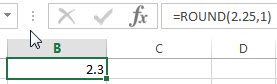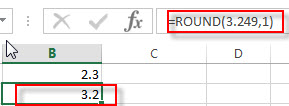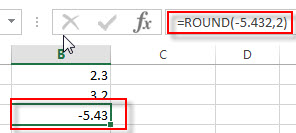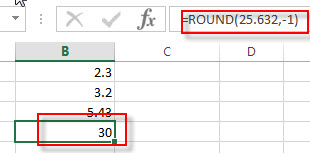# Excel ROUND Function

This post will guide you how to use Excel ROUND function with syntax and examples in Microsoft excel.

### Description

The Excel ROUND function rounds a number to a specified number of digits. You can use the ROUND function to round to the left or right of the decimal point in Excel.

The ROUND function is a build-in function in Microsoft Excel and it is categorized as a Math and Trigonometry Function.

The ROUND function is available in Excel 2016, Excel 2013, Excel 2010, Excel 2007, Excel 2003, Excel XP, Excel 2000, Excel 2011 for Mac.

### Syntax

The syntax of the ROUND function is as below:

`=ROUND (number, num_digits)`

Where the ROUND function arguments are:

• number -This is a required argument. Any real number that you wan to round up.
• num_digits – This is a required argument. The number of digits to which numbe you wnat to round up.

Note:

• If num_digits argument is greater than 0, then the number is rounded to the specified number of digits. So it will round  to the right of the decimal point.
• If num_digits argument is equal to 0, then the number is rounded to the nearest integer.
• If num_digits argument is less than 0, then the number is rounded to the left of the decimal point.
• If you want to round up a number (away from 0), you can use the ROUNDUP function
• If you want to round down a number (toward 0), you can use the ROUNDDOWN function.

### Excel ROUND Function Examples

The below examples will show you how to use Excel ROUND Function to round a given number up or down to a specified number of decimal point.

1# to round a number 2.25 to one decimal places, enter the following formula in Cell B1.

`=ROUND(2.25,1)`2# to round a number 3.249 to one decimal places, enter the following formula in Cell B2.

`=ROUND(3.249,1)`3# to round a number -5.432 to two decimal places, enter the following formula in Cell B3.

`=ROUND(-5.432,2)`4# to round a number 25.632 up to one decimal places to the left of the decimal point, enter the following formula in Cell B4.

`=ROUND(25.632,-1)`### Related Functions

• Excel ROUNDDOWN function
The Excel ROUNDDOWN function round the number down to the specified number of digits. The syntax of the ROUNDDOWN function is as below:=ROUNDDOWN (number, num_digits)…
• Excel ROUNDUP function
The Excel ROUNDUP function rounds the number up to a specified number of decimal places. It will round away from 0.The syntax of the ROUNDUP function is as below:=ROUNDUP (number, num_digits)…
• Excel CEILING Function
The Excel CEILING function returns a given number rounded up to the nearest multiple of a given number of significance. The syntax of the CEILING function is as below:= CEILING (number, significance)…
• Excel CEILING.PRECISE Function
The Excel CEILING.PRECISE function returns a given number rounded up to the nearest multiple of a given number of significance or returns a number that is rounded up to the nearest integer number.The syntax of the CEILING.PRECISE function is as below:=CEILING.PRECISE (number, [significance])…